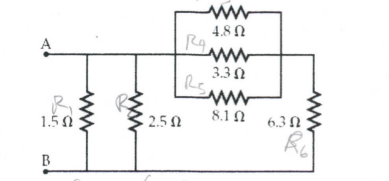Problem: Consider the circuit of resistors below. Determine the equivalent resistance between points A and B.

96% (36 ratings)
Problem Details

Consider the circuit of resistors below.

Determine the equivalent resistance between points A and B.